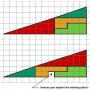# Calculation Speed Test 3There are various subjects in the world including Mathematics. Maths require logic and correction to master it. Somehow, lots of people hate this subject because it involves calculations.

But are you one of them? Or you are the different one? By trying this quiz, you could've checked how good are you in simple Maths. Just do this honestly. The challenge is to complete each question with only 20 seconds limit. This quiz has 20 questions, only YES or NO.

Created by: Matmail

1. 5+83 is lower than 27+52
2. 68+54 is higher than 46+79
3. 68+92 equals 62+98
4. 86+68 equals 49+94
5. The amount of 3's multiplication below 100 are 33 numbers.
6. The amount of numbers between 16 and 32 are 16.
7. The amount of numbers from 18 to 40 are 23.
8. The amount of 4's multiplication between 100 and 200 are 25 numbers.
9. Zero times any number resulting 0.
10. Any number with the power of 0 resulting 0.
11. 5 with the power of 3 equals to 125.
12. 400 is the square form of a negative number.
13. 2 with the power of 4 is higher than 4 with the power of 2.
14. The lowest prime number is 1.
15. 143 is a prime number.
16. The explanation of a prime number is a number that can't be divided by any number except the number itself.
17. 0 is the lowest natural number.
18. The total of natural numbers below 10 is as same as the total of whole numbers below 10.
19. The whole numbers don't contain 0 in the list, but the natural numbers contain.
20. Unlimited can be used to define something unexplainable and unfinishable.

Remember to rate this quiz on the next page!
Rating helps us to know which quizzes are good and which are bad.

What is GotoQuiz? A better kind of quiz site: no pop-ups, no registration requirements, just high-quality quizzes that you can create and share on your social network. Have a look around and see what we're about.